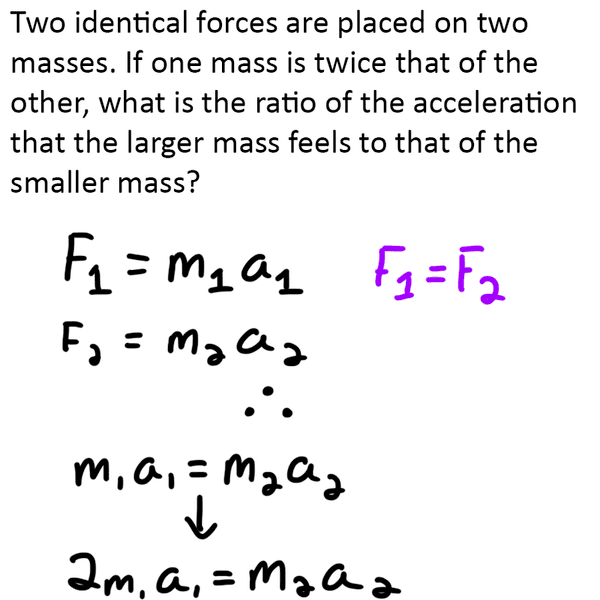# Two identical forces on two different masses...

Member advised to use the homework template for posts in the homework forums.
Here is all the work I have done so far (hopefully it is right). My brain somewhat shuts down when it comes to ratios.PhanthomJay
Homework Helper
Gold Member
no, that equation is not right. Identify which mass is m1 and which is m2, then one mass is twice the other. Correct your equation accordingly, then solve for the ratio.

You wrote:
m1a1 = m2a2
2m1a1 = m2a2

Those two equations contradict each other. Why don't you write an equation for m1 in terms of m2. Then you can substitute for m1 or m2 into the first equation above.

P.S. Welcome to Physics Forums.

Edit: Sorry PhantomJay. I just now saw your post.

You wrote:
m1a1 = m2a2
2m1a1 = m2a2

Those two equations contradict each other. Why don't you write an equation for m1 in terms of m2. Then you can substitute for m1 or m2 into the first equation above.

P.S. Welcome to Physics Forums.

Edit: Sorry PhantomJay. I just now saw your post.

So something more like this then? I got this far, then got confused again as to what kind of ratio I'm supposed to get since I'm not given any values. If I divided by M2, then it would give me (2)A1=A2...so is that saying that the mass that is double the size of the other one is going to accelerate at half the speed of the smaller mass? And would the ratio then be 2:1? Thanks for all your help! :)

EDIT: I'm not sure why my new work isn't showing up...The basics are that I set M1=(2)M2, therefore (2)M2A1=M2A2.

http://imgur.com/a/XdNIG

•TomHart
The basics are that I set M1=(2)M2, therefore (2)M2A1=M2A2.

Edit: Sorry, I read your edit and missed above it. You got the ratio right: 2:1